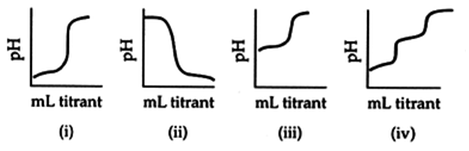# Problem: Examine the titration curves. Which statement is FALSE?A. Curve (i) shows the titration of 50.0 mL of 0.15 M HCl with 0.15 M NaOH.B. Curve (ii) shows the titration of 50.0 mL of 0.15 M NH 4Cl with 0.15 M NaOH.C. Curve (iii) shows the titration of 50.0 mL of 0.15 M CH 3COOH with 0.15 M NaOH.D. Curve (iv) shows the titration of 50.0 mL of 0.15 M H 3PO4 with 0.15 M NaOH.

###### FREE Expert Solution
81% (404 ratings)
###### Problem Details

Examine the titration curves. Which statement is FALSE?

A. Curve (i) shows the titration of 50.0 mL of 0.15 M HCl with 0.15 M NaOH.

B. Curve (ii) shows the titration of 50.0 mL of 0.15 M NH 4Cl with 0.15 M NaOH.

C. Curve (iii) shows the titration of 50.0 mL of 0.15 M CH 3COOH with 0.15 M NaOH.

D. Curve (iv) shows the titration of 50.0 mL of 0.15 M H 3PO4 with 0.15 M NaOH.